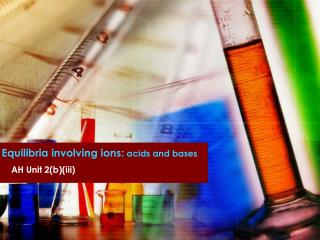DownloadDownload PresentationEquilibria involving ions: acids and bases

Equilibria involving ions: acids and bases

Télécharger la présentationEquilibria involving ions: acids and bases

- - - - - - - - - - - - - - - - - - - - - - - - - - - E N D - - - - - - - - - - - - - - - - - - - - - - - - - - -
Presentation Transcript

1. Equilibria involving ions: acids and bases AH Unit 2(b)(iii)

2. Key question • What is are acids and bases?

3. Arrhenius definition • An acid is a substance that when added to water increases the concentration of H+(aq) ions. HA + (aq)  H+(aq) + A-(aq) • A base is a substance that when added to water increases the concentration of OH-(aq) ions. BOH + (aq)  B+(aq) + OH-(aq)

4. Key question • What are the limitations of these definitions?

5. Bronsted-Lowry definitions • An acid is a proton donor. HA  H++ A- HA + H2O  H3O++ A- • A base is a proton acceptor. B + H+ BH+ B + H3O+  BH+ + H2O

6. Hydronium ion

7. Conjugate acids and bases

8. Ionisation of water • Water is amphoteric. • Can you write an expression for the equilibrium constant?

9. Ionic product of water • Kw has a value of 1 x 10-14 at 25ºC. • Known as the ionic product of water. • Value varies with temperature.

10. pH scale

11. Dissociation of acids This is a measure of the strength of an acid - the larger the value of Ka, the stronger the acid.

12. Relative strengths of acids

13. Calculating pH of weak acids

14. Ionisation of water • The ionisation of water is endothermic. • What will happen to the extent of ionisation of water as temperature increases?

15. Dissociation of bases This is a measure of the strength of a base - the larger the value of Ka, the weaker the base.

16. Buffers

17. Buffer solutions • Is a solution where the pH remains approximately constant when small amounts of acid or bases are added (or when diluted). • Common examples: • blood • sea water • contact lens solution • swimming pools

18. Acid buffers • Consists of a weak acid with one of its salts (of a strong alkali) • e.g. ethanoic acid + sodium ethanoate • This is a weak acid and its conjugate base. • The acid is partially dissociated and equilibrium with its ions. • The salt (conjugate base) is fully ionised.

19. Plenty of Plenty of HA(aq) + H2O(l) H3O+(aq) + A-(aq) Source of H3O+(aq) to replace any removed by addition of extra OH-(aq) Sink that removes extra H3O+(aq)

20. Addition of alkali: Supplies H3O+(aq) ions if any removed in reacting with an added base. • Addition of acid: CH3COONa(s) → Na+(aq) + CH3OO-(aq) The conjugate base removes any added H+(aq)

21. pH of buffer solutions Because by diluting a buffer the concentration of acid and salt will decrease in proportion, dilution will not affect the pH of a buffer solution.

22. Practise

23. Basic buffers • Consist of a weak base with one of its salts (of a strong acid). • Suggest two chemical that could be used to prepare a basic buffer • ammonia • ammonium chloride

24. Indicators

25. Universal indicator

26. Methyl orange

27. Phenolphthalein

28. Indicators • Are weak acids

29. HIn and In- have different colours • Their ratio is dependant on [H3O+] • The colour of an indicator in any given solution therefore depends on the ratio, which in turn is determined by pH

30. The theoretical point at which the colour change occurs is when [HIn] = [In-] • Therefore the colour change occurs when KIn = [H3O+] • pKIn = pH

31. In practice, the colour change is not visible when [HIn] = [In-] • Instead, they usually has to differ by a factor of 10 in order to see it • i.e. when [H+] = KIn± 10 • OR when pH = pKIn ± 1

32. Example For an indicator which is red in the unionised form (HIn) and blue in the ionic form (In-), it will appear: • Completely red when [HIn] > 10 x [In-] • Completely blue when [In-] > 10 x [HIn] This means that a colour change takes place typically over 2 pH units.

33. Choice of indicator • Colour change must occur as close to the equivalence point as possible. • Equivalence point – the point at which all of the acid has been exactly “neutralised” by all of the alkali. • Does this always occur at pH 7? • Ideally, the equivalence point will equal the end point.

34. Rapid initial rise

35. The pH at which an indicator changes colour depends on the value of the pKa for that indicator. • A good indicator will change colour on addition of one or two drops of the acid or base, corresponding to the steepest part of the titration curve.

36. Methyl red

37. Phenolphthalein